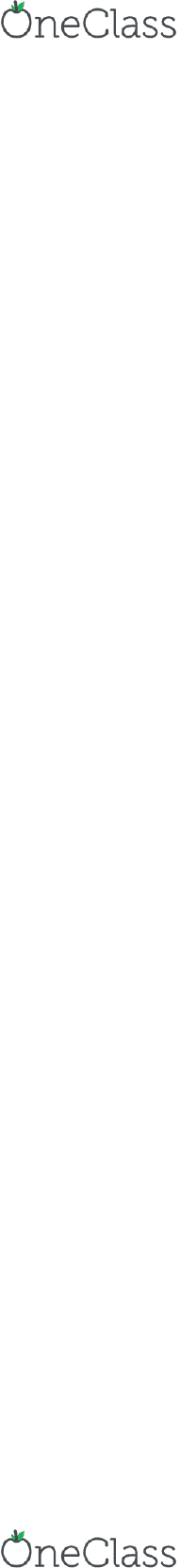Study Guides (400,000)
US (230,000)
Harvard (700)
MATH (400)
math (1)
Midterm

# Mathematics 21a Study Guide - Midterm Guide: Cone

Department
Mathematics
Course Code
Mathematics 21a
Professor
math
Study Guide
Midterm

This preview shows page 1. to view the full 5 pages of the document.Notes: Important formulas to be kept in mind:
Parameters of a Cone: Radius of the base (r ), Height of the cone (h ) and Slant
Height of a Cone (l )
Volume of a Cone = \frac{1}{3} \pi r^2 h
Curved Surface area of a Cone = \pi r l
Total Surface area of a Cone = \pi r^2+ \pi r l
Question 1: Find the volume of a cone whose slant height is 17 \ cm and radius
of base is 8 \ cm .
Volume of a Cone = \frac{1}{3} \pi r^2 h
l = 17 \ cm, \ r = 8 \ cm
Therefore h = \sqrt{l^2 - r^2} = \sqrt{17^2-8^2} = 15 \ cm
Therefore Volume = \frac{1}{3} \times \frac{22}{7} \times (8)^2 \times (15) =
1005.71 \ cm^3
\\
Question 2: The curved surface area of a cone is 12320 \ cm^2 . If the radius of
its base is 56 \ cm , find its height.
Curved surface area of the cone = 12320 \ cm^2
r = 56 \ cm
\pi r l = 12320 \Rightarrow l = \frac{12320}{\pi . 56} = 70 \ cm
Therefore h = \sqrt{l^2 - r^2} = \sqrt{70^2-56^2} = 1542 \ cm
\\
Question 3: The circumference of the base of a 12 \ m high conical tent is 66 \
m . Find the volume of the air contained in it.
h = 12 \ m
Circumference of the base = 66 \ m
Therefore 2 \pi r = 66 \Rightarrow r = \frac{33}{\pi}
Volume of a Cone = \frac{1}{3} \pi r^2 h
= \frac{1}{3} \times \pi \times (\frac{33}{\pi})^2 \times (12) = 1386 \ m^3
\\
Question 4: The radius and the height of a right circular cone are in the ratio
5:12 and its volume is 2512 \ cm^3 . Find the radius and slant height of the
find more resources at oneclass.com
find more resources at oneclass.com

Unlock to view full version

Only page 1 are available for preview. Some parts have been intentionally blurred.cone. (Take \pi = 3.14 )
Given: Radius and the height of a right circular cone are in the ratio 5:12
Let r = 5x and h = 12 x
Volume = 2512 \ cm^3
Therefore: \frac{1}{3} \pi r^2 h = 2512
\Rightarrow \frac{1}{3} \times 3.14 \times (5x)^2 \times (12x) = 2512
\Rightarrow x^3 = 8 \ or \ x = 2
Therefore Radius = 5 (2) = 10 \ cm and Height = 12(2) = 24 \ cm
\\
Question 5: Two right circular cones X and, Y are made. X having three times
the radius of Y and Y having half the volume of X . Calculate the ratio
between the heights of X and Y .
Let the radius of cone Y is x . Therefore the radius of cone X = 3x .
Let the height of cone Y = h_y and the height of cone X = h_x
Given: V_y = \frac{1}{2} V_x
\Rightarrow \frac{1}{3} \pi (x)^2 (h_y) = \frac{1}{2} \{ \frac{1}{3} \pi (3x)^2
(h_x) \}
\Rightarrow \frac{h_x}{h_y} = \frac{x^2}{(3x)^2}
\Rightarrow \frac{h_x}{h_y} = \frac{2}{9}
\\
Question 6: The diameters of two cones are equal. If their slant heights are in
the ratio 5 : 4 , find the ratio of their curved surface areas.
Let the radius of Cone 1 and Cone 2 = x
Let the slant height of the cones be l_1 and l_2 respectively.
Curved Surface Area of a cone = \pi r l
Therefore
\frac{Curved \ Surface \ Area \ of \ Cone \ 1}{Curved \ Surface \ Area \ of \
Cone \ 2} = \frac{\pi \times x \times l_1}{\pi \times x \times l_2} = \frac{5}
{4}
\\
Question 7: There are two cones. The curved surface area of one is twice that of
the other. The slant height of the latter is twice that of the former. Find the